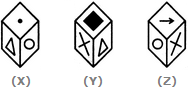# Non Verbal Reasoning - Cubes and Dice - Discussion

### Discussion :: Cubes and Dice - Section 2 (Q.No.31)

31.

A cube has six different symbols drawn over its six faces. The symbols are dot, circle, triangle, square, cross and arrow. Three different positions of the cube are shown in figures X, Y, and Z.

Which symbol is opposite the dot?[A]. Circle [B]. Triangle [C]. Arrow [D]. Cross

Explanation:

From figures X and Y, we conclude that dot, circle, square and cross lie adjacent to the triangle. Therefore, the arrow must lie opposite the triangle. From figures X and Z, we conclude that dot, triangle, arrow and cross lie adjacent to the circle. Therefore, the square must lie opposite the circle. Thus, the arrow lies opposite the triangle, the square lies opposite the circle and consequently, the cross lies opposite the dot.

As analysed above, the cross lies opposite the dot.

 Vikrant Singh said: (Apr 28, 2018) It should be triangle or cross.

 Vyshnavi said: (Oct 9, 2020) Agree, It should be cross.